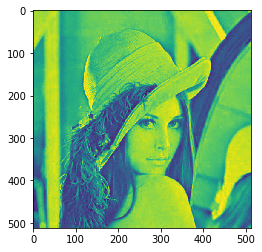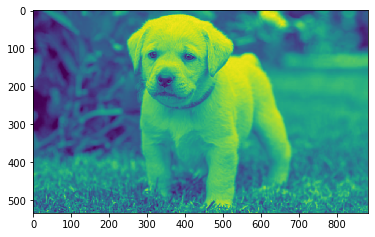Related Articles

# Mahotas – Eccentricity of Image

• Last Updated : 02 Sep, 2021

In this article we will see how we can get the eccentricity of the image in mahotas. Eccentricity measures the shortest length of the paths from a given vertex v to reach any other vertex w of a connected graph. Computed for every vertex v it transforms the connectivity structure of the graph into a set of values. For a connected region of a digital image it is defined through its neighbourhood graph and the given metric.

`mahotas.demos.load('lena')`

Below is the lena imageIn order to do this we will use mahotas.features.eccentricity( method
Syntax : mahotas.features.eccentricity(img)
Argument : It takes image object as argument
Return : It returns float value

Note : Input image should be filtered or should be loaded as grey
In order to filter the image we will take the image object which is numpy.ndarray and filter it with the help of indexing, below is the command to do this

`image = image[:, :, 0]`

Below is the implementation

## Python3

 `# importing required libraries``import` `mahotas``import` `mahotas.demos``from` `pylab ``import` `gray, imshow, show``import` `numpy as np``import` `matplotlib.pyplot as plt``  ` `# loading image``img ``=` `mahotas.demos.load(``'lena'``)``  ` `# filtering image``img ``=` `img.``max``(``2``)` `print``(``"Image"``)``  ` `# showing image``imshow(img)``show()` `# computing eccentricity value``value ``=` `mahotas.features.eccentricity(img)`` `  `# showing value``print``(``"Eccentricity value = "` `+` `str``(value))`

Output :

`Image``Eccentricity value = 0.0`

Another example

## Python3

 `# importing required libraries``import` `mahotas``import` `numpy as np``from` `pylab ``import` `gray, imshow, show``import` `os``import` `matplotlib.pyplot as plt`` ` `# loading image``img ``=` `mahotas.imread(``'dog_image.png'``)`  `# filtering image``img ``=` `img[:, :, ``0``]``  ` `print``(``"Image"``)``  ` `# showing image``imshow(img)``show()` `# computing eccentricity value``value ``=` `mahotas.features.eccentricity(img)`` `  `# showing value``print``(``"Eccentricity value = "` `+` `str``(value))`

Output :

`Image``Eccentricity value = 0.7950893156644899`

Attention geek! Strengthen your foundations with the Python Programming Foundation Course and learn the basics.

To begin with, your interview preparations Enhance your Data Structures concepts with the Python DS Course. And to begin with your Machine Learning Journey, join the Machine Learning – Basic Level Course

My Personal Notes arrow_drop_up